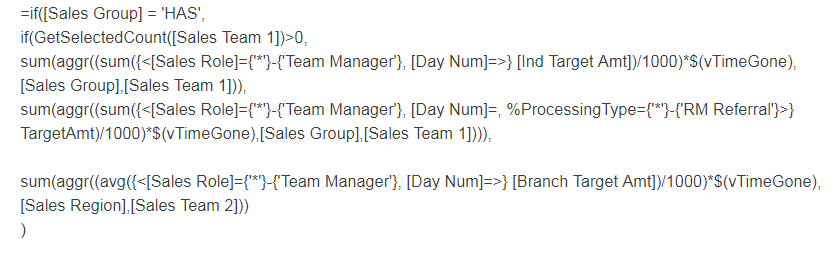# QlikView App Development

Discussion Board for collaboration related to QlikView App Development.

Announcements
Save the Date: QlikWorld Online, June 24-25, 2020. Free global virtual event for data integration and data analytic gurus. Register Today
Highlighted
New Contributor III

## Pivot Table Total

Hi Guys,

My pivot table does not seems not sum properly for MTD Target and Target:The calculation for MTD target is:

=if([Sales Group] = 'HAS',

if(GetSelectedCount([Sales Team 1])>0,

sum(aggr((sum({<[Sales Role]={'*'}-{'Team Manager'}, [Day Num]=>} [Ind Target Amt])/1000)*\$(vTimeGone),[Sales Group],[Sales Team 1])),

sum(aggr((sum({<[Sales Role]={'*'}-{'Team Manager'}, [Day Num]=, %ProcessingType={'*'}-{'RM Referral'}>} TargetAmt)/1000)*\$(vTimeGone),[Sales Group],[Sales Team 1]))),

sum(aggr((avg({<[Sales Role]={'*'}-{'Team Manager'}, [Day Num]=>} [Branch Target Amt])/1000)*\$(vTimeGone),[Sales Region],[Sales Team 2]))

)

and if i switched the first if condition to:

=if([Sales Group] <> 'Franchise',

if(GetSelectedCount([Sales Team 1])>0,

sum(aggr((sum({<[Sales Role]={'*'}-{'Team Manager'}, [Day Num]=>} [Ind Target Amt])/1000)*\$(vTimeGone),[Sales Group],[Sales Team 1])),

sum(aggr((sum({<[Sales Role]={'*'}-{'Team Manager'}, [Day Num]=, %ProcessingType={'*'}-{'RM Referral'}>} TargetAmt)/1000)*\$(vTimeGone),[Sales Group],[Sales Team 1]))),

sum(aggr((avg({<[Sales Role]={'*'}-{'Team Manager'}, [Day Num]=>} [Branch Target Amt])/1000)*\$(vTimeGone),[Sales Region],[Sales Team 2]))

)

The total will be 66,200 instead of 1760.

1 Solution

Accepted Solutions
HighlightedMVP

## Re: Pivot Table TotalYou need an overarching Aggr() function over the complete expression... something like this

=Sum(Aggr(

if([Sales Group] = 'HAS',

if(GetSelectedCount([Sales Team 1])>0,

sum(aggr((sum({<[Sales Role]={'*'}-{'Team Manager'}, [Day Num]=>} [Ind Target Amt])/1000)*\$(vTimeGone),[Sales Group],[Sales Team 1])),

sum(aggr((sum({<[Sales Role]={'*'}-{'Team Manager'}, [Day Num]=, %ProcessingType={'*'}-{'RM Referral'}>} TargetAmt)/1000)*\$(vTimeGone),[Sales Group],[Sales Team 1]))),

sum(aggr((avg({<[Sales Role]={'*'}-{'Team Manager'}, [Day Num]=>} [Branch Target Amt])/1000)*\$(vTimeGone),[Sales Region],[Sales Team 2]))

), .....))

5 Replies
HighlightedMVP

## Re: Pivot Table Total

Highlighted
New Contributor III

## Re: Pivot Table Total

Hi Sunny,

Thanks!

But i did include the aggr function. just that the parameters are different:

sum(aggr((sum({<[Sales Role]={'*'}-{'Team Manager'}, [Day Num]=, %ProcessingType={'*'}-{'RM Referral'}>} TargetAmt)/1000)*\$(vTimeGone),[Sales Group],[Sales Team 1]))),

sum(aggr((avg({<[Sales Role]={'*'}-{'Team Manager'}, [Day Num]=>} [Branch Target Amt])/1000)*\$(vTimeGone),[Sales Region],[Sales Team 2]))

Is that the reason why?

HighlightedMVP

## Re: Pivot Table Total

Regards,

Great dreamer's dreams never fulfilled, they are always transcended.
HighlightedMVP

## Re: Pivot Table TotalYou need an overarching Aggr() function over the complete expression... something like this

=Sum(Aggr(

if([Sales Group] = 'HAS',

if(GetSelectedCount([Sales Team 1])>0,

sum(aggr((sum({<[Sales Role]={'*'}-{'Team Manager'}, [Day Num]=>} [Ind Target Amt])/1000)*\$(vTimeGone),[Sales Group],[Sales Team 1])),

sum(aggr((sum({<[Sales Role]={'*'}-{'Team Manager'}, [Day Num]=, %ProcessingType={'*'}-{'RM Referral'}>} TargetAmt)/1000)*\$(vTimeGone),[Sales Group],[Sales Team 1]))),

sum(aggr((avg({<[Sales Role]={'*'}-{'Team Manager'}, [Day Num]=>} [Branch Target Amt])/1000)*\$(vTimeGone),[Sales Region],[Sales Team 2]))

), .....))

Highlighted
New Contributor III

## Re: Pivot Table Total

Thank you very much! Works